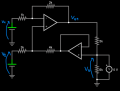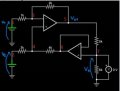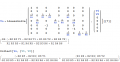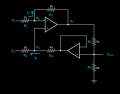# Op-Amp circuit with both positive and negative feedback

#### RitterTree

Joined Dec 18, 2022
23
Hi everyone, first time posting.
For the past couple of days I've been struggling to come up with a solution to this problem: I'm asked to write the output voltage $${V_{0}}$$ as function of the input voltages $${V_{1}}$$ and $${V_{2}}$$: $${V_{0}}=f({V_{1},{V_{2}}})$$.So far I've tried approaching the problem with the superposition principle as follows:

$$KCL)$$ $$\frac{{V_{1}}-V^{-}}{{R_{1}}}=\frac{V^{-}-{V_{01}}}{{R_{2}}}$$
$$Given$$ $${V_{2}} = 0$$ $$then$$ $${V^{+}}=\frac{{R_{3}}}{{R_{3}}+{R_{4}}}{V_{0}}={V^{-}}$$
$$therefore$$ $$\frac{{V_{1}}}{{R_{1}}}-\frac{{R_{3}}}{{R_{1}}}\frac{1}{{R_{3}}+{R_{4}}}{V_{0}}=\frac{{R_{3}}}{{R_{2}}}\frac{1}{{R_{3}}+{R_{4}}}{V_{0}}-\frac{{V_{01}}}{{R_{2}}}$$

But I'm not able to find a clean relationship between $${V_{01}}$$ and $${V_{0}}$$ so as to be able to write $${V_{0}}=f({V_{1}})$$.
Then I would have done the same thing given $${V_{1}} = 0$$ and added the two results together. Am I missing something?

#### WBahn

Joined Mar 31, 2012
29,165
Isn't V_0 nothing more than V_01 put through a voltage divider?

•RitterTree

#### RitterTree

Joined Dec 18, 2022
23
Isn't V_0 nothing more than V_01 put through a voltage divider?
$${V_{0}}=\frac{{R_{6}}}{R_{5}+{R_{6}}}{{V_{01}}}$$ ?

#### WBahn

Joined Mar 31, 2012
29,165
R_5 and R_6 are undefined reference designators. If you are going to use R_x to refer to the x kΩ resistor, then you need to say that.

But, assuming this is the case, then yes.

Whatever voltage appears at V_0 produces a current through R_5 and R_6, which are in series as long as it is assumed that no current flows into the opamp inputs, which is an assumption you've already made to get as far as you did. V_0 is then just the voltage that that current produces when flowing through R_6.

•RitterTree

#### RitterTree

Joined Dec 18, 2022
23
R_5 and R_6 are undefined reference designators. If you are going to use R_x to refer to the x kΩ resistor, then you need to say that.
Yes, I meant it that way, I'm sorry for not clarifying.

Thank you for the answer! It's clear now! I don't know how I didn't see it before..

#### RitterTree

Joined Dec 18, 2022
23
Continuing...

$$with$$ $${V_{0}}=\frac{{R_{6}}}{R_{5}+{R_{6}}}{{V_{01}}}$$
$$then$$ $${V_{01}}=\frac{{R_{5}}+{R_{6}}}{R_{6}}{{V_{0}}}$$
$$then$$ $$\frac{{V_{1}}}{{R_{1}}}=\left( \frac{{R_{3}}}{{R_{3}}+{R_{4}}} \right)\left( \frac{1}{{R_{1}}}+\frac{1}{{R_{2}}} \right){V_{0}}-\frac{1}{{R_{2}}}\left( \frac{{R_{5}}+{R_{6}}}{{R_{6}}} \right){V_{0}}$$
$$then$$ $$\frac{{V_{1}}}{{R_{1}}}=\left[ \left( \frac{{R_{3}}}{{R_{3}}+{R_{4}}} \right)\left( \frac{1}{{R_{1}}}+\frac{1}{{R_{2}}} \right)-\frac{1}{{R_{2}}}\left( \frac{{R_{5}}+{R_{6}}}{{R_{6}}} \right)\right]{V_{0}}$$
$$then$$ $$\frac{{V_{1}}}{{R_{1}}}=\left[ \frac{{R_{3}}{R_{6}}\left( {R_{1}}+{R_{2}} \right)-{R_{1}}\left( {R_{3}}+{R_{4}} \right)\left( {R_{5}}+{R_{6}} \right)}{{R_{1}}{R_{2}}{R_{6}}\left( {R_{3}}+{R_{4}} \right)} \right]{V_{0}}$$
$$therefore\, {V_{0}}=\left[ \frac{{R_{2}}{R_{6}}\left( {R_{3}}+{R_{4}} \right)}{{R_{3}}{R_{6}}\left( {R_{1}}+{R_{2}} \right)-{R_{1}}\left( {R_{3}}+{R_{4}} \right)\left( {R_{5}}+{R_{6}} \right)} \right]{V_{1}}$$

Now shutting down the other voltage source:

$$given\, {V_{1}}=0$$
$$KCL) \, -\frac{{V^{-}}}{{R_{1}}}=\frac{{V^{-}}-{V_{01}}}{{R_{2}}}$$
$$with\, {V_{01}}=\frac{{R_{5}}+{R_{6}}}{R_{6}}{{V_{0}}}$$
$$then\, {V^{-}}=\left( \frac{{R_{5}}+{R_{6}}}{{R_{6}}} \frac{{R_{1}}}{{R_{1}}+{R_{2}}}\right){V_{0}}$$

$$with\, Millman: {V^{+}}=\frac{\frac{{V_{2}}}{{R_{3}}}+\frac{{V_{0}}}{{R_{4}}}}{\frac{1}{{R_{3}}}+\frac{1}{{R_{4}}}}$$

$$with\, {V^{+}}={V^{-}}$$
$$then\, \left( \frac{{R_{5}}+{R_{6}}}{{R_{6}}} \frac{{R_{1}}}{{R_{1}}+{R_{2}}}\right){V_{0}} =\frac{{R_{4}}}{{R_{3}}+{R_{4}}}{V_{2}}+\frac{{R_{3}}}{{R_{3}}+{R_{4}}}{V_{0}}$$
$$then\, \left( \frac{{R_{5}}+{R_{6}}}{{R_{6}}} \frac{{R_{1}}}{{R_{1}}+{R_{2}}}-\frac{{R_{3}}}{{R_{3}}+{R_{4}}}\right){V_{0}} =\frac{{R_{4}}}{{R_{3}}+{R_{4}}}{V_{2}}$$
$$therefore\, {V_{0}}=\left[ \frac{{R_{4}}{R_{6}}\left( {R_{1}}+{R_{2}} \right)}{{R_{1}}\left( {R_{3}}+{R_{4}} \right)\left( {R_{5}}+{R_{6}} \right)-{R_{3}}{R_{6}}\left( {R_{1}}+{R_{2}} \right)} \right]{V_{2}}$$

Putting the two results together:

$${V_{0}}=\left[ \frac{{R_{2}}{R_{6}}\left( {R_{3}}+{R_{4}} \right)}{{R_{3}}{R_{6}}\left( {R_{1}}+{R_{2}} \right)-{R_{1}}\left( {R_{3}}+{R_{4}} \right)\left( {R_{5}}+{R_{6}} \right)} \right]{V_{1}} +\left[ \frac{{R_{4}}{R_{6}}\left( {R_{1}}+{R_{2}} \right)}{{R_{1}}\left( {R_{3}}+{R_{4}} \right)\left( {R_{5}}+{R_{6}} \right)-{R_{3}}{R_{6}}\left( {R_{1}}+{R_{2}} \right)} \right]{V_{2}}$$

Is it (at least conceptually) correct? Are there any smarter and faster ways to solve the problem?

#### The Electrician

Joined Oct 9, 2007
2,936
Continuing...

$$with$$ $${V_{0}}=\frac{{R_{6}}}{R_{5}+{R_{6}}}{{V_{01}}}$$
$$then$$ $${V_{01}}=\frac{{R_{5}}+{R_{6}}}{R_{6}}{{V_{0}}}$$
$$then$$ $$\frac{{V_{1}}}{{R_{1}}}=\left( \frac{{R_{3}}}{{R_{3}}+{R_{4}}} \right)\left( \frac{1}{{R_{1}}}+\frac{1}{{R_{2}}} \right){V_{0}}-\frac{1}{{R_{2}}}\left( \frac{{R_{5}}+{R_{6}}}{{R_{6}}} \right){V_{0}}$$
$$then$$ $$\frac{{V_{1}}}{{R_{1}}}=\left[ \left( \frac{{R_{3}}}{{R_{3}}+{R_{4}}} \right)\left( \frac{1}{{R_{1}}}+\frac{1}{{R_{2}}} \right)-\frac{1}{{R_{2}}}\left( \frac{{R_{5}}+{R_{6}}}{{R_{6}}} \right)\right]{V_{0}}$$
$$then$$ $$\frac{{V_{1}}}{{R_{1}}}=\left[ \frac{{R_{3}}{R_{6}}\left( {R_{1}}+{R_{2}} \right)-{R_{1}}\left( {R_{3}}+{R_{4}} \right)\left( {R_{5}}+{R_{6}} \right)}{{R_{1}}{R_{2}}{R_{6}}\left( {R_{3}}+{R_{4}} \right)} \right]{V_{0}}$$
$$therefore\, {V_{0}}=\left[ \frac{{R_{2}}{R_{6}}\left( {R_{3}}+{R_{4}} \right)}{{R_{3}}{R_{6}}\left( {R_{1}}+{R_{2}} \right)-{R_{1}}\left( {R_{3}}+{R_{4}} \right)\left( {R_{5}}+{R_{6}} \right)} \right]{V_{1}}$$

Now shutting down the other voltage source:

$$given\, {V_{1}}=0$$
$$KCL) \, -\frac{{V^{-}}}{{R_{1}}}=\frac{{V^{-}}-{V_{01}}}{{R_{2}}}$$
$$with\, {V_{01}}=\frac{{R_{5}}+{R_{6}}}{R_{6}}{{V_{0}}}$$
$$then\, {V^{-}}=\left( \frac{{R_{5}}+{R_{6}}}{{R_{6}}} \frac{{R_{1}}}{{R_{1}}+{R_{2}}}\right){V_{0}}$$

$$with\, Millman: {V^{+}}=\frac{\frac{{V_{2}}}{{R_{3}}}+\frac{{V_{0}}}{{R_{4}}}}{\frac{1}{{R_{3}}}+\frac{1}{{R_{4}}}}$$

$$with\, {V^{+}}={V^{-}}$$
$$then\, \left( \frac{{R_{5}}+{R_{6}}}{{R_{6}}} \frac{{R_{1}}}{{R_{1}}+{R_{2}}}\right){V_{0}} =\frac{{R_{4}}}{{R_{3}}+{R_{4}}}{V_{2}}+\frac{{R_{3}}}{{R_{3}}+{R_{4}}}{V_{0}}$$
$$then\, \left( \frac{{R_{5}}+{R_{6}}}{{R_{6}}} \frac{{R_{1}}}{{R_{1}}+{R_{2}}}-\frac{{R_{3}}}{{R_{3}}+{R_{4}}}\right){V_{0}} =\frac{{R_{4}}}{{R_{3}}+{R_{4}}}{V_{2}}$$
$$therefore\, {V_{0}}=\left[ \frac{{R_{4}}{R_{6}}\left( {R_{1}}+{R_{2}} \right)}{{R_{1}}\left( {R_{3}}+{R_{4}} \right)\left( {R_{5}}+{R_{6}} \right)-{R_{3}}{R_{6}}\left( {R_{1}}+{R_{2}} \right)} \right]{V_{2}}$$

Putting the two results together:

$${V_{0}}=\left[ \frac{{R_{2}}{R_{6}}\left( {R_{3}}+{R_{4}} \right)}{{R_{3}}{R_{6}}\left( {R_{1}}+{R_{2}} \right)-{R_{1}}\left( {R_{3}}+{R_{4}} \right)\left( {R_{5}}+{R_{6}} \right)} \right]{V_{1}} +\left[ \frac{{R_{4}}{R_{6}}\left( {R_{1}}+{R_{2}} \right)}{{R_{1}}\left( {R_{3}}+{R_{4}} \right)\left( {R_{5}}+{R_{6}} \right)-{R_{3}}{R_{6}}\left( {R_{1}}+{R_{2}} \right)} \right]{V_{2}}$$

Is it (at least conceptually) correct? Are there any smarter and faster ways to solve the problem?
Your result is correct. As to the question "Are there any smarter and faster ways to solve the problem?" I'll show how I solved the problem and you can form your own opinion as to whether it's smarter and faster.

I used nodal analysis and solved the equations using linear algebra techniques. I wanted the computer to do all the algebra so I selected 7 nodes. This is more than absolutely necessary, but results in all the algebra being done by computer.•RitterTree

#### RitterTree

Joined Dec 18, 2022
23
I used nodal analysis and solved the equations using linear algebra techniques. I wanted the computer to do all the algebra so I selected 7 nodes. This is more than absolutely necessary, but results in all the algebra being done by computer.
I'm not very software savvy and I've never properly looked into nodal analysis. Can it be done the same way efficiently by hand too? I'm preparing for a pen and paper exam so I'm not allowed to use computers.

#### The Electrician

Joined Oct 9, 2007
2,936
I'm not very software savvy and I've never properly looked into nodal analysis. Can it be done the same way efficiently by hand too? I'm preparing for a pen and paper exam so I'm not allowed to use computers.

The only reason I used linear algebra is to avoid doing the algebra by hand. It's still necessary to know how to set up the nodal equations. Having done so, they can be solved by hand just like it was done before computers.And, of course what you did in this thread gave you the correct solution, so that's a method you could use for the exam.

You say you haven't looked into nodal analysis. What is the exam testing? Is it your knowledge of some particular skill in solving networks? Is it just testing your general knowledge of solving neworks by whatever method you choose?

#### WBahn

Joined Mar 31, 2012
29,165
Are there any smarter and faster ways to solve the problem?
This is how I would probably have approached it on an exam:

First, annotate the diagram with the symbols you are going to use (and add others as needed):Make note of key relationships and assumptions. This will help whomever is reviewing your work (your supervisor, client, or in this case, the grader) to see what your thinking is.

Assuming Ideal Op Amps: No current into any input, and inputs are virtual shorts.

$$V_B \; = \; V_A \\ V_{out} \; = \; V_C \frac{R_6}{R_5 \; + \; R_6} \\ \\ I_A = \frac{V_1 \; - \; V_A}{R_1} \\ I_B \; = \; \frac{V_{out} \; - \; V_2}{R_3 \; + \; R_4} \\ \\ V_B \; = \; V_2 \; + \; I_B R_3 \\ V_C \; = \; V_A \; - \; I_A R_2$$

At this point, you've captured all of the EE of the problem. Everything else is just math. A good grader would award the bulk of the points for this much and, if prior exams have indicated that the grader believes strongly in partial credit, then at this point I would move on to get other problems to a similar point and then come back and finish them off as time permits, starting with the ones that look like they will take the least time to wrap up.

•Jerry-Hat-Trick and RitterTree

#### RitterTree

Joined Dec 18, 2022
23
You say you haven't looked into nodal analysis. What is the exam testing? Is it your knowledge of some particular skill in solving networks? Is it just testing your general knowledge of solving neworks by whatever method you choose?
It's a fundamentals of electronics exam. It's about everything that has to do with the analysis of circuits with diodes, MOSFETs and Op-Amps like amplifiers, filters, oscillators, converters and CMOS logic. They take for granted that you already know how to solve circuits because we've already done exams about the very basics of electronics.

More than not having looked into nodal analysis I'm just not well versed in it because I've been using mesh analysis more.

#### RitterTree

Joined Dec 18, 2022
23
At this point, you've captured all of the EE of the problem. Everything else is just math. A good grader would award the bulk of the points for this much and, if prior exams have indicated that the grader believes strongly in partial credit, then at this point I would move on to get other problems to a similar point and then come back and finish them off as time permits, starting with the ones that look like they will take the least time to wrap up.
That is very good advice, thanks! I always waste time doing math even if I'm not sure I'm going in the right direction.

#### MrAl

Joined Jun 17, 2014
10,606
Hi everyone, first time posting.
For the past couple of days I've been struggling to come up with a solution to this problem: I'm asked to write the output voltage $${V_{0}}$$ as function of the input voltages $${V_{1}}$$ and $${V_{2}}$$: $${V_{0}}=f({V_{1},{V_{2}}})$$.
View attachment 295665
So far I've tried approaching the problem with the superposition principle as follows:

<snip>

But I'm not able to find a clean relationship between $${V_{01}}$$ and $${V_{0}}$$ so as to be able to write $${V_{0}}=f({V_{1}})$$.
Then I would have done the same thing given $${V_{1}} = 0$$ and added the two results together. Am I missing something?

Hello there,

Refer to the attached drawing where i have added three labels va, vb, and vc.
I will also refer to the resistor values as Rv where v is the value in kilohms.

The simplest way is to try to use what we already know and see if we can apply that to the circuit at hand.

Starting with Op2, we can see that is a simple voltage follower, so any voltage at vc will appear at the output.
Op1 forms a non-inverting amplifier with va, and that also has a non-zero input that gets inverted (v1).

Because of the Op1 topology we can immediately write the output expression:
vb=va*(1+G1)-v1*G1 [this is the superposition of the two input voltages and the respective gains]
where G1 is the gain formed from R2 and R1.

Now since vc is just vb times another gain G2, we have:
vc=vb*G2

We also have va as the superposition of vc (remember Op2 is a voltage follower so Vout=vc) and v2 giving us:
va=v2*G4+vc*G3

Now all that needs to be done is to solve those three equations simultaneously, then substitute the gains
with the proper resistor expressions for those gains.

Solving simultaneously, we get one expression for each node va, vb, and vc:
va=-(v2*G4-v1*G1*G2*G3)/(G1*G2*G3+G2*G3-1)
vb=-(v2*G1*G4+v2*G4-v1*G1)/(G1*G2*G3+G2*G3-1)
vc=-(G2*(v2*G1*G4+v2*G4-v1*G1))/(G1*G2*G3+G2*G3-1)

and since vc is the most interesting, we factor that a little nicer and get:
vc=(G2*(v2*G1*G4+v2*G4-v1*G1))/(1-G1*G2*G3-G2*G3)

All that remains now is to substitute the resistor expressions in for the four gains G1 to G4 and simplify a bit, and then we have the result.
See if you can replace the four gains with the proper resistor expressions and get the same result as you got before.

One last note:
Because there is positive feedback in this circuit it should be checked for stability and/or for latch up.

#### Attachments

Last edited:
•RitterTree

#### RitterTree

Joined Dec 18, 2022
23
Op1 forms a non-inverting amplifier with va, and that also has a non-zero input that gets inverted (v1).

Because of the Op1 topology we can immediately write the output expression:
vb=va*(1+G1)-v1*G1 [this is the superposition of the two input voltages and the respective gains]
where G1 is the gain formed from R2 and R1.

Now since vc is just vb times another gain G2, we have:
vc=vb*G2

We also have va as the superposition of vc (remember Op2 is a voltage follower so Vout=vc) and v2 giving us:
va=v2*G4+vc*G3

Now all that needs to be done is to solve those three equations simultaneously, then substitute the gains
with the proper resistor expressions for those gains.

Solving simultaneously, we get one expression for each node va, vb, and vc:
va=-(v2*G4-v1*G1*G2*G3)/(G1*G2*G3+G2*G3-1)
vb=-(v2*G1*G4+v2*G4-v1*G1)/(G1*G2*G3+G2*G3-1)
vc=-(G2*(v2*G1*G4+v2*G4-v1*G1))/(G1*G2*G3+G2*G3-1)

and since vc is the most interesting, we factor that a little nicer and get:
vc=(G2*(v2*G1*G4+v2*G4-v1*G1))/(1-G1*G2*G3-G2*G3)

All that remains now is to substitute the resistor expressions in for the four gains G1 to G4 and simplify a bit, and then we have the result.
Thank you for the answer, this is so clean! How can one develop the eye to see things so modularly and be able not to waste time with long calculations like you did?

#### The Electrician

Joined Oct 9, 2007
2,936
Simplifying MrAl's method (do substitutions first):

Starting with: vb=va*(1+G1)-v1*G1

Take note of: va=v2*G4+vc*G3

Substitute that in for va and get: vb=(v2*G4+vc*G3)*(1+G1)-v1*G1

Substitute this in for vb in vc=vb*G2 and get: vc=((v2*G4+vc*G3)*(1+G1)-v1*G1)*G2

Now we need only solve one equation in vc to get an expression for vc which, with some algebra, can be
whipped into the desired final form V0=vc=f(v1,v2).

No matter what method you use, in the end if you have to do it all by hand, there will be a fair amount
of algebra to slog through.

•RitterTree

#### MrAl

Joined Jun 17, 2014
10,606
Thank you for the answer, this is so clean! How can one develop the eye to see things so modularly and be able not to waste time with long calculations like you did?
Hello there,

It's very interesting that you used the word "modularly" because i was meaning to describe this technique as being a "modular" technique.

The idea is very simple. You look at the circuit and try to find sub circuits that you are already familiar with and have inputs and outputs that can be isolated in a manner that does not change the basic modular form of the sub circuits.
The two examples for this circuit were the voltage follower and the non inverting amplifier with an included input that gets inverted.
If you know how a voltage follower works, you can understand how Op2 affects the two nodes it is associated with.
If you already know how the non inverting amplifier works, and you know how an inverting amplifier works, you can use superposition to know how the non inverting amplifier with included input that gets inverted works. You just then just need to know how resistors affect gains: one for the gain of the amplifier Op1, and another for a voltage divider.

There will be cases where this doesn't work because the modules can not be isolated from each other so easily. It may take some experience with these circuits to figure this out, and even then there may be novel cases that are not as easy to recognize. That's when we really have to turn to a full-blown analysis technique such as nodal.
If we do use nodal and we have to use the same circuit over and over again for different applications with other resistor values, we may be able to develop those modular techniques for that particular circuit which would then help when the newer applications come along.

Another small note:
Sometimes you can get by with just using the expression with all the gains G1 to G4 and just describing these gains separately such as:
G1=R2/R1

and just leave the last expression with all the G's in it as is. This helps to keep the final expression a little neater.
This also helps a great deal in a program to calculate the expression. First calculate all the gains, then use those numerical values to substitute into the final expression with all the G's.

Last edited:
•RitterTree

#### RitterTree

Joined Dec 18, 2022
23
You look at the circuit and try to find sub circuits that you are already familiar with and have inputs and outputs that can be isolated in a manner that does not change the basic modular form of the sub circuits.
There will be cases where this doesn't work because the modules can not be isolated from each other so easily. It may take some experience with these circuits to figure this out, and even then there may be novel cases that are not as easy to recognize.
When it comes to pattern recognition, lately, I'm hesitant to isolate sub circuits because I'm afraid that I'll let something slip up and end up making the whole analysis conceptually wrong (it cost me greatly in the past), so I always end up going with full blown mesh analysis on the whole circuit until something interesting comes up and I can start putting things together. It's highly inefficient and very risky to invest a lot of time doing math without having a big picture in mind but I'm just not experienced enough. Will have to work on that in the future.

I wonder how much it is a matter of being accustomed to well known configurations versus just being good at putting things together.

#### MrAl

Joined Jun 17, 2014
10,606
When it comes to pattern recognition, lately, I'm hesitant to isolate sub circuits because I'm afraid that I'll let something slip up and end up making the whole analysis conceptually wrong (it cost me greatly in the past), so I always end up going with full blown mesh analysis on the whole circuit until something interesting comes up and I can start putting things together. It's highly inefficient and very risky to invest a lot of time doing math without having a big picture in mind but I'm just not experienced enough. Will have to work on that in the future.

I wonder how much it is a matter of being accustomed to well known configurations versus just being good at putting things together.
Hi,

The more circuits you analyze the more you start to see shortcuts, but of course you have to be careful, and for anything really important you should have two ways to analyze it in case you make a mistake, and mistakes are always lurking. Spice is great for this, it's like a second opinion.

•RitterTree